Courses

# Test: Permutation & Combination- 4

## 15 Questions MCQ Test UPSC Prelims Paper 2 CSAT - Quant, Verbal & Decision Making | Test: Permutation & Combination- 4

Description
This mock test of Test: Permutation & Combination- 4 for UPSC helps you for every UPSC entrance exam. This contains 15 Multiple Choice Questions for UPSC Test: Permutation & Combination- 4 (mcq) to study with solutions a complete question bank. The solved questions answers in this Test: Permutation & Combination- 4 quiz give you a good mix of easy questions and tough questions. UPSC students definitely take this Test: Permutation & Combination- 4 exercise for a better result in the exam. You can find other Test: Permutation & Combination- 4 extra questions, long questions & short questions for UPSC on EduRev as well by searching above.
QUESTION: 1

### Direction for questions 1 to 2 : Read passage below and solve the questions based on it. Tn a horticultural show, a participant arranged a total of 1000 fruits of four types in a long straight line He first placed one apple, then two mangoes, three oranges, four custard apples, five apples, six mangoes, seven oranges, eight custard apples and so on (with apples followed by mangoes, oranges, custard apples in that order) uplo the 1000th fruit. What was the 1000th fruit?

Solution:

Fruits are ordered like 1. Apple 2. Mangoes 3 Oranges 4. Custard apple Fruits arc being pul up first one. then two, then three, then four and so oil.

1 + 2 + 3 + ... +44 = 990
So, the 45th fruit will be the 100th fruit. Since we are having a set of four different types of fruits, 45th will be Apple.

QUESTION: 2

### Direction for questions 1 to 2: Read passage below and solve the questions based on it. In a horticultural show, a participant arranged a total of 1000 fruits of four types in a long straight line He first placed one apple, then two mangoes, three oranges, four custard apples, five apples, six mangoes, seven oranges, eight custard apples and so on (with apples followed by mangoes, oranges, custard apples in that order) uplo the 1000th fruit. Q. What was the position of the 100th Mango?

Solution:

Fruits are ordered like 1. Apple 2. Mangoes 3 Oranges 4. Custard apple Fruits arc being pul up first one. then two, then three, then four and so oil.

Mangoes will come like 2, 6, 10, 14 etc. 100th mango will come 2 + 6 + 10 + 14 + 18 + 22 + 26 + 2= 100
100th mango will come in its 8th turn.
Before that apple must have got its 8th turn, orange and custard apple must have got their 7th turn.
Total apples displayed till now = 1 + 5 + 9 + ... + 29= 120
Total oranges displayed till now = 3 + 7+11 + ... +27=105
Total custard apples displayed till now = 4 + 8+ 12 + ... +28= 112
So, total fruits displayed till now (other than mangoes) = 337
So, the position of the 100th mango = 437

QUESTION: 3

### Consider S = (1, 2, 3,... 10). In how many ways two numbers from S can be selected so that the sum of the numbers selected is a double digit number?

Solution:

If the sum is a two-digit number, then both the values simultaneously cannot be less than 5. Now start making the sets by taking different values greater than or equal to atleast one of the numbers.

QUESTION: 4

Find the number of non-negative integer solutions to the system of equations a + b + c + d + e = 20 and a + b + c = 5 is

Solution:

Given a + b + c + d+ e = 20 ...(1)

a + b + c = 5 ... (2)

Given, system of equations is equivalent to a + b + c = 5 ...(3)
and d+ e = 15

Number non-negative integral solutions of equation (3)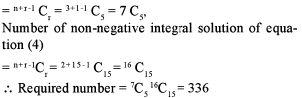QUESTION: 5

In a test of 10 multiple choice questions of one correct answer, each having 4 alternative answers, then the number of ways to put ticks at random for the answers to all the questions is

Solution:

Each of the questions can be answered in 4 ways. So, 10 questions can be answered in 410 ways.

QUESTION: 6

IfN be an element of the set A= {1,2,3,5,6,10,15,30} and P, Q and R are integers such that PQR = N, then the number of positive integral solutions of PQR = N, is

Solution:

This is nothing but the application of the concept of number of factors (see Number System).

QUESTION: 7

Number of ways in which n distinct things can be distributed among n persons so that atleast one person does not get anything is 232. Find n.

Solution:

nn - n! = 232. Use options now.

QUESTION: 8

The number of employees in a nationalised bank in a small town is 10, out of which 4 are female and the rest male. A committee of 5 is to be formed. If m be the number of ways to form such a committee in which there is atleast one female employee and n be the number of ways to form such a committee whichincludes at least two male employees, then find the ratio m:n.

Solution: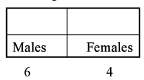Now take different cases to fulfill the conditions given.

QUESTION: 9

In how many ways 4 men and 4 women can be seated in a row so that men and women are alternate?

Solution: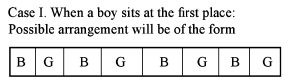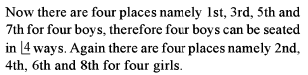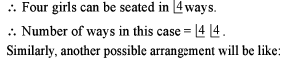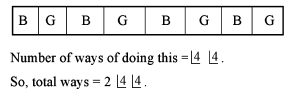QUESTION: 10

In a chess tournament every person played one game with every other person in the group. The total number of games that men played between themselves exceeded those played by men with women by 18. If there were 4 women in the tournament, in all how many games were played in the tournament?

Solution:

MC2 - MC1 wC1 = 18, where M and W are the number of men and women respectively.

QUESTION: 11

A dinner menu is to be designed out of 5 different starters, 6 identical main courses and 4 distinct desserts. In how many ways menu be designed such that there is atleast one of each of the starters, main courses and desserts?

Solution:
QUESTION: 12

A tea party is arranged for 16 people along the two sides of a long table with 8 chairs on each side. Four men wish to sit on one particular side and two on the other side. In how many ways can they be seated?

Solution:

Four persons have chosen to sit on one particular side (assume side A) and two persons on the other side (assume side B). So, we are supposed to select four persons for side A from the remaining 10 persons and remaining six persons will be sitting on side B. Number of ways 4 persons can be selected from 10 persons = 10C4

Number of ways 6 persons can be selected from the remaining 6 persons = 6C6

Number of ways 8 persons can be arranged on side A = 8!
Number of ways 8 persons can be arranged on side B = 8!
Total number of ways = 10C4 x 6C6 x 8! x 8!

QUESTION: 13

There are 12 intermediate stations between two places A and B. In how many ways can a train be made to stop at 4 of these 12 intermediate stations that no two stations are consecutive?

Solution:

L et S 1, S2, . . . , S8 denote the stations w here the train does not stop. The four stations where the train stops should be at any four of the nine places indicated by cross.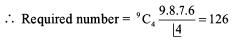QUESTION: 14

If the number of ways in which n different things can be distributed among n persons so that at least one person does not get any thing is 232 then what is the value of vfl

Solution:

Go through the options using formula.

QUESTION: 15

How many three-digit numbers with distinct digits can be formed such that the product of the digits is the cube of a positive integer?

Solution: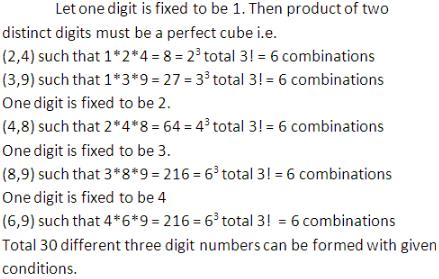Track your progress, build streaks, highlight & save important lessons and more!

### Similar Content### Related tests